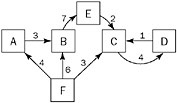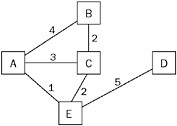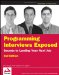Graphs

Graphs are more complicated than trees. Like trees, they consist of nodes with children. (A tree is actually a type of graph.) Unlike trees, a node can have multiple “parents,” possibly creating a loop (a cycle). In addition, the links between nodes, as opposed to the nodes themselves, may have values or weights. These links are called edges because they may contain more information than just a pointer. In a graph, edges can be one way or two way. A graph with one-way edges is called a directed graph. A graph with only two-way pointers is called an undirected graph. A directed graph is shown in Figure 5-4, and an undirected graph is shown in Figure 5-5.Figure 5-4Figure 5-5

Graphs are commonly used to model real-world problems that are difficult to model with other data structures. For example, a directed graph could represent the aqueducts connecting cities (since water only flows one way). You might use such a graph to help you find the fastest way to get water from city A to city D. An undirected graph can represent something complicated, like a series of relays in signal transmission.

Unlike trees, there are many ways to represent graph data structures in code. The choice of representation is often determined by the algorithm being implemented. Graphs are often used in real-world programming, but graph problems are difficult to solve in the time allotted for an interview, so the preceding overview of graph definitions should be sufficient.Programming Interviews Exposed: Secrets to Landing Your Next Job, 2nd Edition (Programmer to Programmer)
ISBN: 047012167X
EAN: 2147483647
Year: 2007
Pages: 94

Similar book on Amazon